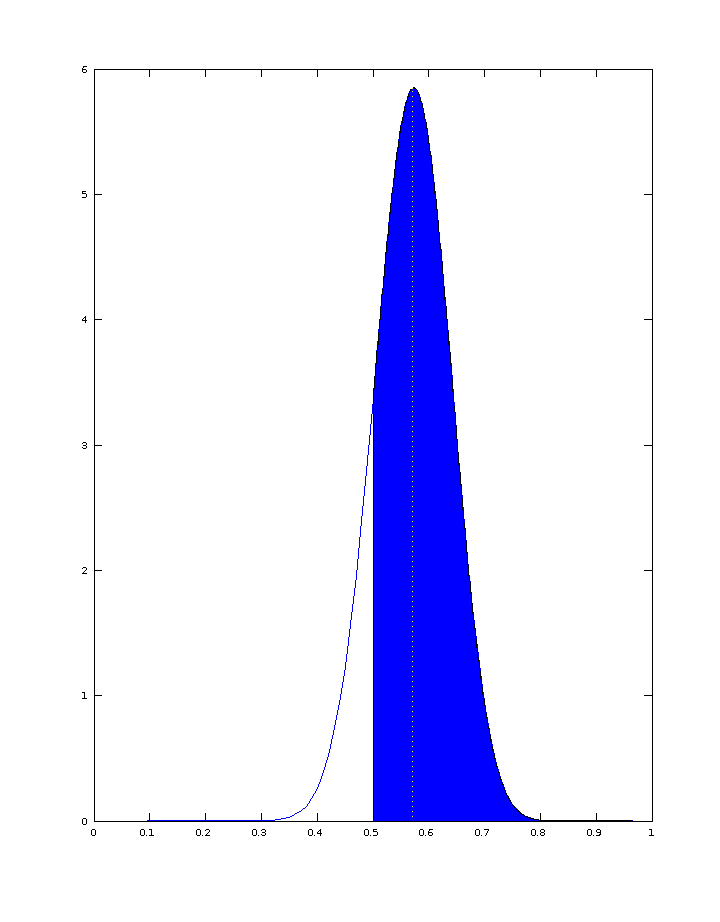## Sunday, 17 August 2014

### Modelling the #Indyref outcome probabilities

Given the latest poll-of-poll results (courtesy of @WhatScotsThink on Twitter) and the betting exchange implied probabilities (courtesy of @neiledwardlovat on Twitter) pertaining to the upcoming Scottish Independence Referendum, I thought it would be interesting to combine these sources of information into (approximate) probability density functions for YES and for NO, respectively.

### Meaningful criteria for the approximate probability distributions

In advance of the actual referendum on 18 September 2014 (which will settle the matter once and for all), assume that the proportion of voters who will vote YES can be modelled as a random variable, denoted Y. Likewise, for the proportion of voters who will vote NO, denoted N. Although the true probability density functions for these random variables are fundamentally unknown, meaningful estimates of the distributions can be derived given that they should reasonably satisfy the following criteria:

• The Expected Value (approximated by the Mean or Average) of the given distribution should reflect the result of the aggregate of all the official polls conducted to date. Note: this criterion is only justifiable if the polls can be considered as truly representative of the actual full voting population. This is, of course, a highly debatable assertion, one extreme view being that "opinion polls are meaningless". But for the sake of current argument, the assertion that the polls are indeed representative, will be considered as valid.
• The integral of the distribution (or "area under the probability density function" or "cumulative density function (cdf)") evaluated over the range from 0.5 to 1 (50% to 100%) should correspond to the implied probabilities ("chance of YES winning", and "chance of NO winning", respectively) from the betting exchange data. It could be viewed that this is a considerably less justifiable criterion than the previous, but on the basis that "the bookies are seldom wrong", it seems like quite a reasonable assertion. After all, the betting exchange data is "crowd-sourced" in that it reflects the combined "beliefs" of many thousands of punters, i.e.,  potential voters. Also, there is no other readily available source of such information, with the exception of the polling data itself (utilised in the previous criterion). However, modelling probability distributions on just the polling data would lead to much narrower distributions (i.e., suggesting considerably less uncertainty) than implied from the betting exchange data. Or put another way, the betting exchange data in effect incorporates the (potentially real) broader uncertainties than captured in the polling data alone.

### The Beta Distribution

Since the probability distributions for Y and N are fundamentally unknown, we are free to build models which meet the criteria presented above. A good candidate is the Beta Distribution which is often used for the purpose at hand, i.e., to devise a meaningful probability distribution for election outcomes involving two choices. One of the benefits of using the Beta Distribution  is that it is formally (structurally) similar to the Binomial Distribution which is widely used for modelling polling results. Taken together, these two distributions can be combined via Bayesian Inference to provide an updated-distribution-after-most-recent-poll which, is itself a Beta Distribution. This useful and interesting aspect will not be pursued at present.

The Beta Distribution has two parameters, denoted A ("alpha") and B ("beta"). The numerical size of these determines the sharpness/certainty (or broadness/uncertainty) with large numerical values representing narrow distributions (i.e., with more certainty), and smaller numerical values representing broad distributions (i.e., with more uncertainty). Also, if A and B are numerically equal, the distribution is symmetric about a Mean value of 0.5 (50%). Unequal values for  A and B allow for skewed (non-symmetric) distributions with Mean values different from 0.5.

By trial-and-error, it is straightforward to determine the parameters (A, B) for the Beta Distributions which satisfy the criteria described earlier for both YES and NO. The results of these parameterisations are presented in the distributions below.

### Probability Density Function (pdf) for YESProbability Density Function (pdf) of a Beta Distribution parameterised (with A=21, B=27.89) to represent the random variable Y (or YES) based on polling data and betting exchange data as of 17 August 2014.  The Mean of the distribution (indicated by the dotted vertical line) is 0.43 corresponding to the poll-of-polls data which suggests that 43% will vote YES. The cumulative density evaluated from 0.5 to 1 (i.e., the area of the blue shaded region)  representing the probability that Y will exceed 0.5 i.e., that YES will win, is 0.15  in accordance with the 15% implied probability from the betting exchange data.

### Probability Density Function (pdf) for NOProbability Density Function (pdf) of a Beta Distribution parameterised (with A=30.3, B=22.81) to represent the random variable N (or NO) based on polling data and betting exchange data as of 17 August 2014.  The Mean of the distribution (indicated by the dotted vertical line) is 0.57 corresponding to the poll-of-polls data which suggests that 57% will vote NO. The cumulative density evaluated from 0.5 to 1 (i.e., the area of the blue shaded region)  representing the probability that N will exceed 0.5 i.e., that NO will win, is 0.86  in accordance with the 86% implied probability from the betting exchange data.

### What are these good for ?

I would suggest that these approximate distributions, derived in accordance with the criteria presented, might be useful for anybody interested in exploring the statistics of #Indyref.  For example, if you are inclined to build a Bayesian Inference model for monitoring how the probabilities evolve following successive polls in the days leading up to 18 September 2014, these distributions might serve as usable "priors". Then again, all must be taken with a (large?) pinch of salt, since the assumptions and data used for deriving the distributions, by their very nature, may turn out to be non-valid :-)